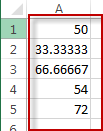# Multiply or Divide a Range of Cells with by a Number

This post will guide you how to multiply or divide a range of cells by a number in Excel. How do I multiply or divide entire range by the same number with VBA Macro in Excel.

Assuming that you have a list of data (A1:A5) that you want to multiply or divide the selected range of cells by a specified number (6). How to achieve it. You can use the Paste Special Feature to divide or multiply the selected range of cells by a number. Or you can use an excel VBA Macro to achieve the same result quickly.

## Divide range by a number with Paste Special Feature

#1 insert a divisor number in a blank cell, such as: C1. And then press Ctrl +C shortcut.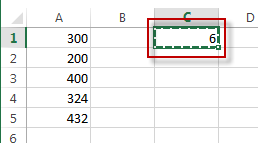#2 select the range of cells that you want to divide values by the divisor number entered in the above steps, and Right click on the selected range, select Paste Special from the pop up menu list. The Paste Special dialog will open.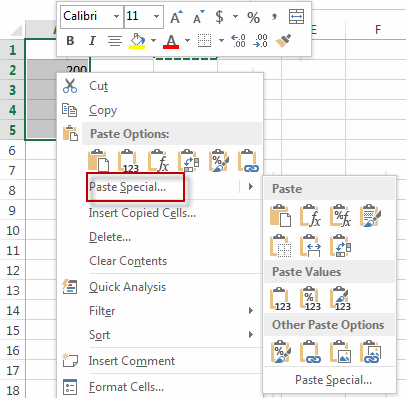#3 select the Multiply or Divide radio button, then click OK button.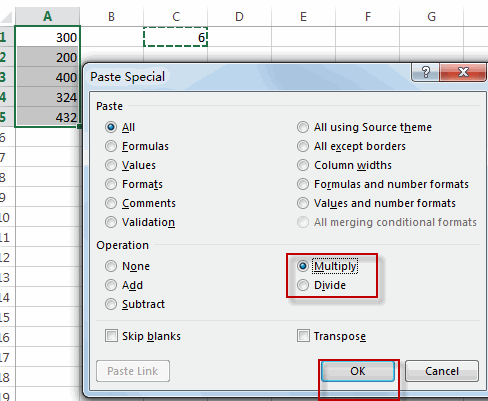#4 let’s see the result.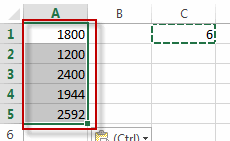## Divide range by a number with VBA

You can also use an Excel VBA macro to divide a range of cells by a number or multiply a range of cells. You just need to do the following steps:

#1 open your excel workbook and then click on “Visual Basic” command under DEVELOPER Tab, or just press “ALT+F11” shortcut.#2 then the “Visual Basic Editor” window will appear.

#3 click “Insert” ->”Module” to create a new module#4 paste the below VBA code into the code window. Then clicking “Save” button.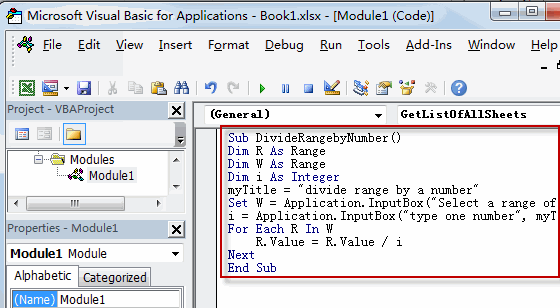```Sub DivideRangebyNumber()
Dim R As Range
Dim W As Range
Dim i As Integer
myTitle = "divide range by a number"
Set W = Application.Selection
Set W = Application.InputBox("Select a range of cells that you want to divide", myTitle, W.Address, Type:=8)
i = Application.InputBox("type one number", myTitle, Type:=1)
For Each R In W
R.Value = R.Value / i
Next
End Sub```

If you want to multiply a range of cells by a number, you can use the below VBA code:

```Sub MultiplyRangebyNumber()
Dim R As Range
Dim W As Range
Dim i As Integer
myTitle = "multiply range by a number"
Set W = Application.Selection
Set W = Application.InputBox("Select a range of cells that you want to multipy", myTitle, W.Address, Type:=8)
i = Application.InputBox("type one number", myTitle, Type:=1)
For Each R In W
R.Value = R.Value * i
Next
End Sub```

#5 back to the current worksheet, then run the above excel macro. Click Run button.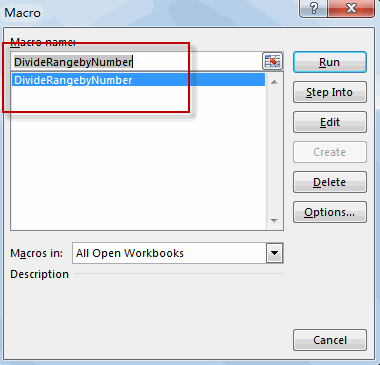#6 select a range of cells that you want to divide or multipy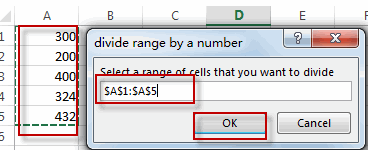#7 type one number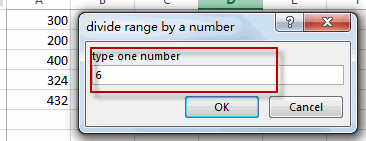#8 Let’s see the result: